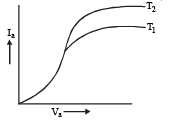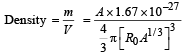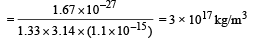Courses

# True/False of Modern Physics, Past year Questions JEE Advance, Class 12, Physics JEE Notes | EduRev

## JEE : True/False of Modern Physics, Past year Questions JEE Advance, Class 12, Physics JEE Notes | EduRev

The document True/False of Modern Physics, Past year Questions JEE Advance, Class 12, Physics JEE Notes | EduRev is a part of the JEE Course Class 12 Physics 35 Years JEE Mains &Advance Past year Paper.
All you need of JEE at this link: JEE

True/False

Q.1. The kinetic energy of photoelectrons emitted by a photosensitive surface depends on the intensity of the incident  radiation.      (1981- 2 Marks)

Ans. F

Solution. For photoelectric effect

hv – hv0 = (K.E.)max

where  h = Planck’s constt.

v0 = Threshold frequency

⇒  (K.E.)max ∝ n

K.E. does not depend on the intensity of incident radiation.

Q.2. In a photoelectric emission process the maximum energy of the photo-electrons increases with increasing intensity of the incident light.        (1986 - 3 Marks)

Ans. F

Solution. (K.E.)max = hv – hv0 ⇒ (K.E.)max ∝ v

Thus maximum kinetic energy is proportional to frequency and not intensity.

Q.3. For a diode the variation of its anode current Ia with the anode voltage Va at two different cathode temperatures T1 and T2  is shown in the figure. The temperature T2  is greater than T1.      (1986 - 3 Marks)Ans. T

Solution. Note : When the cathode temperature is higher, then more number of electrons will be emitted which in turn will increase the anode current.

Q.4. The order of magnitude of the density of nuclear matter is 104 kg m–2.        (1989 - 2 Marks)

Ans. F

Solution.where A = mass number.

Note : The order of nuclear density is 1017 kg/m3.

Offer running on EduRev: Apply code STAYHOME200 to get INR 200 off on our premium plan EduRev Infinity!

42 docs|19 tests

,

,

,

,

,

,

,

,

,

,

,

,

,

,

,

,

,

,

,

,

,

,

,

,

,

,

,

,

,

,

;